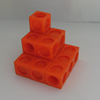#### You may also like### Picture Story

Can you see how this picture illustrates the formula for the sum of the first six cube numbers?### The Root of the Problem

Find the sum of this series of surds.### Sums of Powers - A Festive Story

A story for students about adding powers of integers - with a festive twist.

# Slick Summing

##### Age 14 to 16Challenge Level

In the video below, Charlie works out $1+2+3+4+5+6+7+8+9+10$.

Can you see how his method works?

How could you adapt his method to work out the following sums?

$1 + 2 + 3 + \dots + 19 + 20$

$1 + 2 + 3 + \dots + 99 + 100$

$40 + 41 + 42 + \dots + 99 + 100$

Can Charlie's method be adapted to sum sequences that don't go up in ones?

$1 + 3 + 5 + \dots + 17 + 19$

$2 + 4 + 6 + \dots + 18 + 20$

$42 + 44 + 46 + \dots + 98 + 100$

Can you find an expression for the following sum?
$1 + 2 + 3 + \dots + (n - 1) + n$

Notes and Background

If you enjoyed this problem you may be interested to read the article Clever Carl, which tells the story of the young Gauss working on sums like the ones in this problem.